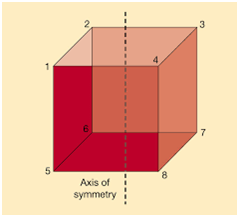# Axis of Symmetry Formula

Axis of symmetry is a line that divides an object into two equal halves, thereby creating a mirror-like reflection of either side of the object. The word symmetry implies balance. Symmetry can be applied to various contexts and situations. Symmetry is a key concept in geometry which cuts the figure into two halves that are exact reflections of each other, as shown in the figure given below.For a parabola, the axis of symmetry is given by the formula,
$\large x = \frac{-b}{2a} for \: Quadratic \: Equation,\: y = ax^{2}+bx+c$
Where,

a and b are coefficients of x2 and x respectively.
c is a constant term.

Example 1:

Find the axis of symmetry of the graph of y =

$$\begin{array}{l}x^{2}\end{array}$$
−6x+5, using the formula.

Solution:

Given,

y = x2 – 6x + 5

For a quadratic function in standard form, y = a

$$\begin{array}{l}x^{2}\end{array}$$
+bx+c, the axis of symmetry is a vertical line,  x =
$$\begin{array}{l}\frac{-b}{2a}\end{array}$$
Here, a = 1, b = −6 and c = 5

Substituting the values of a and b,

x = -(-6)/2(1)

= 6/2

= 3
Therefore, the axis of symmetry is x = 3.

Example 2:

Find the axis of symmetry of the graph of y = 2x2 + 8x – 3, using the formula.

Solution:

Given,

y = 2x2 + 8x – 3

Comparing the given equation with the standard form y = ax2 + bx + c,

a = 2, b = 8, c = -3

And the axis of symmetry is a vertical line; x = -b/2a

Substituting the values of a and b,

x = -8/2(2)

= -8/4

= -2

Therefore, the axis of symmetry is x = -2.

Example 3:

If the axis of symmetry of the equation y = px2 – 12x – 5 is 2, then find the value of p.

Solution:

Given,

y = px2 – 12x – 5

Axis of symmetry is x = 2

For a quadratic function in standard form, y=a

$$\begin{array}{l}x^{2}\end{array}$$
+bx+c, the axis of symmetry is a vertical line,  x =
$$\begin{array}{l}\frac{-b}{2a}\end{array}$$

Here, a = p, b = -12, c = -5

According to the given,

-b/2a = 2

-(-12)/2p = 2

12 = 4p

12/4 = p

Therefore, p = 3